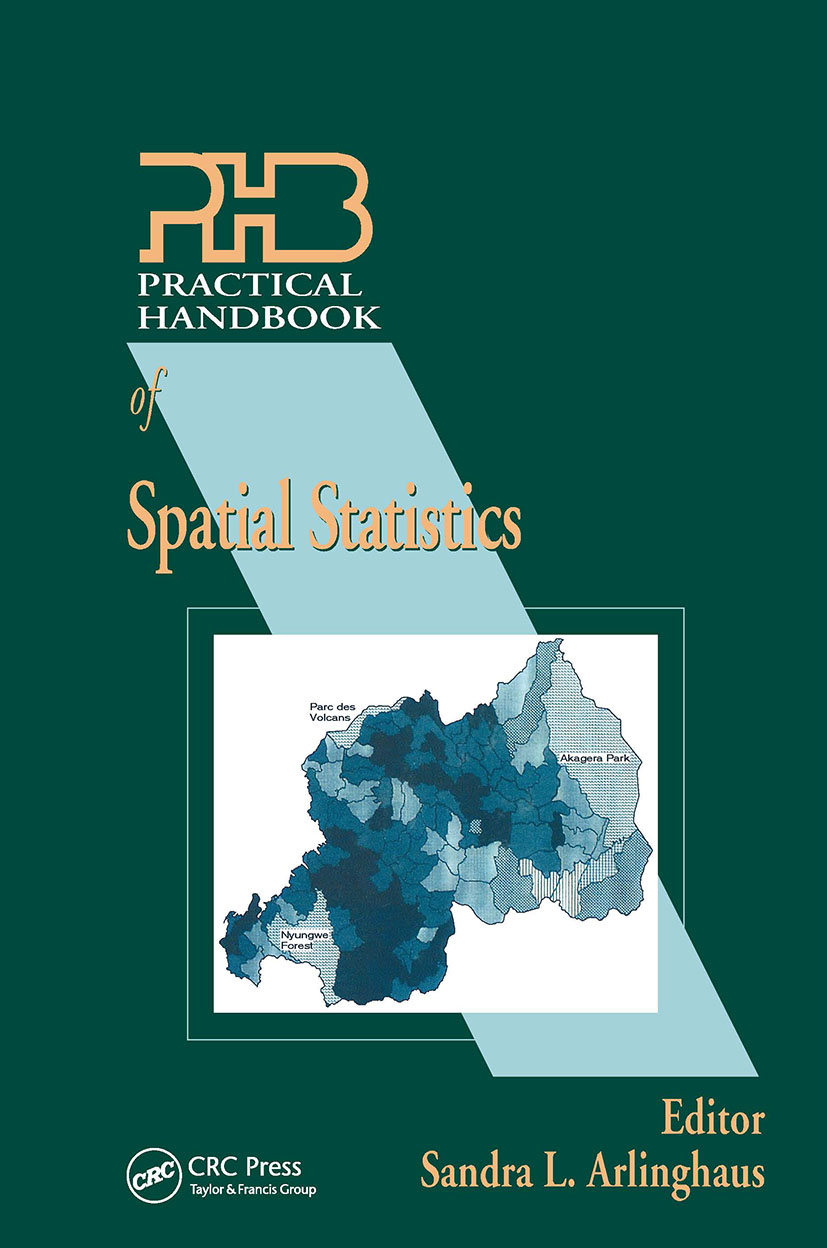Practical Handbook of Spatial Statistics

1st Edition

CRC Press

336 pages

Purchasing Options:\$ = USD
Hardback: 9780849301322
pub: 1995-10-05
SAVE ~\$35.00
\$175.00
\$140.00
x

FREE Standard Shipping!

Description

The guidance and special techniques provided in this handbook will allow you to understand and use complex spatial statistical techniques. You will learn how to apply proper spatial analysis techniques and why they are generally different from conventional statistical analyses. Clear and concise information on weighting, aggregation effects, sampling, spatial statistics and GIS, and visualization of spatial dependence is provided. Discussions on specific applications using actual data sets fill obvious gaps in the literature, and coverage of critical research frontiers allows readers to explore current areas of active research.

Reviews

"Practical Handbook of Spatial Statistics does achieve its objective of providing a handbook that practitioners can pick up and use immediately…most people who work with environmental data will enjoy reading about these applications and seeing how spatial statistical models can be used in practice."

-JASA, March 1999

Introduction: The Need for Spatial Statistics, D.A. Griffith

Components of Geographic Information and Analysis

Background: The Importance of Locational Information

Background: Statistical Estimator Properties

Organization of the Book

Summary

References

Visualization of Spatial Dependence: An Elementary View of Spatial Autocorrelation, I.R. Vasiliev

Editorial Note

Introduction

The Spatial Mean and Other Basic Concepts

Spatial Autocorrelation

Map Complexity

Map Representations of Changes in Space and Time

Summary: Rules-of-Thumb for Spatial Autocorrelation

References

Spatial Sampling, S.V. Stehman and W.S. Overton

Introduction

Spatial Universes and Populations

Sampling Fundamentals

Sampling a Continuous Universe

Sampling Spatially Distributed Objects via Areal Samples of the Continuous Universe

Inference in Spatial Sampling

Applications of Spatial Sampling

Empirical Evaluation of Sampling Strategies

Summary

References

Some Guidelines for Specifying the Geopraphic Weights Matrix Contained in Spatial Statistical Models, D.A. Griffith

Introduction

Background

Evaluation Criteria

Rules-of-Thumb Implications

References

Aggregation Effects in Geo-Referenced Data, D.W.S. Wong

Spatial Dependency of Spatial Data Analysis

Source of the MAUP: Spatial Dependence and the Averaging Process

General Impacts of the MAUP on Spatial Data

Approaches to "Solving" the MAUP

Guidelines for Analyzing Data From Different Scales

Conclusions

References

Implementing Spatial Statistics on Parallel Computers, B. Li

Introduction

A Brief Introduction to Parallel Processing

Software Models for Parallel Processing

Parallel Implementations

Performance

Summary

References

Appendix I: Test Statistics for Spatial Autocorrelation Coefficients

Appendix II: Source Code

Spatial Statistics and GIS Applied to Internal Migration in Rwanda, Central Africa, D.G. Brown

Introduction

Study Area

Database Description

GIS Data Management

Mapping Residuals

Spatial Statistical Model

Conclusions

References

Spatial Statistical Modeling of Regional Fertility Rates: A Case Study of He-Nan Province, China, H.M. Feng

Introduction

Preliminary Considerations of the Spatial Statistical Application

The Dataset and the Model Specification

Explicit Variables

A Classical Linear Regression Model of Explicit Variables

In Search of a Spatial Pattern

Interpretation and Conclusions

References

Appendix I: Description of Data Set

Appendix II: Maps

Appendix III: Scatter-Plots

Spatial Statistical/Econometric Versions of Simple Urban Population Density Models, D.A. Griffith and A. Can

Introduction and Background

The Selected Metropolitan Landscapes

Preliminaries for Estimating the Autoregressive Model

The Estimated Population Density Models

Implementation Findings

References

Spatial Statistics for Analysis of Variance of Agronomic Field Trials, D.S. Long

The Example Data Set

Goals of the Case Study

The Autoregressive Response Model

Calculating the Moran Coefficient

Calculating the Necessary Eigenvalues

Estimating the Jacobian Term

Estimating an Autoregressive Response Model

Comparison of AR-based ANOVA and Conventional ANOVA

Conclusions

Acknowledgments

References

Index